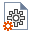Join one of our upcoming courses taking place around the world! Explore now

# Capacity Ratio

Capacity ratio is a term used here to determine the structural Demand/Capacity of the reinforced concrete section. The Demand is the resultant factored bending moment obtained from the analysis of the pile at a certain level and Capacity is the structural factored calculated bending moment capacity at an axial load level equal to the same applied at that section.

The capacity ratio is calculated over the pile length at all of its reinforced concrete sections. To calculate the Capacity Ratio:

1. Select Home > Project Settings2. Go to the Interaction diagrams tab.
3. Select the Capacity Ratio checkbox.